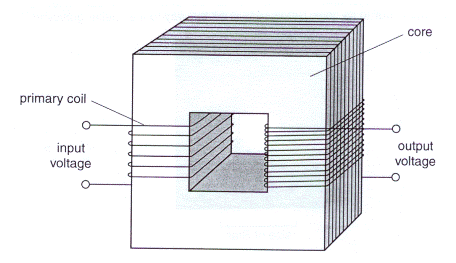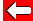# GCSE Physics Exam

Source: OCR June 2003. Reproduced with permission.

2. The diagram shows a simple step-up transformer.(a) For safety, a low voltage is needed for a child's train set.

How can this transformer be changed so that a mains voltage can be reduced to a low output voltage? [2+1]

(b) Alternating current must be used for a transformer to work.

(i) What is alternating current? 

(ii) Why must an alternating current be used? 

(c) (i) Another transformer is being used to step down the voltage from 240 V to 12 V.

The primary coil of this transformer has 4000 turns.

Calculate how many turns are needed on the secondary coil.

You must show how you work our your answer.

number of turns on secondary coil = .......... 

(ii) The output of this transformer is connected to a radio.

State and explain how the output current to the radio compares with the input current to the transformer.

Assume the transformer is 100% efficient. 

[Total: 10]

Hints and Answers coming soon!Go back a pageElectricity MenuNext question